Absolute Value
Exponents
Order of Operations
Distributive Property
Percent
Rate and Ratio
100

Find the absolute value of:

|-10|100

When you see the following expression, what is the big number called and what is the little number on top of it called?

24

100

Simplify the following expression: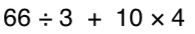100

-4 + -5100

8(4+2) = ?

48

100

What is 25% of 80

20

100

Balia knows that 6 cups of rice will feed 15 people.  How many cups of rice are needed to feed 135 people?

54 cups

200

What is greater:

|-6| or |3|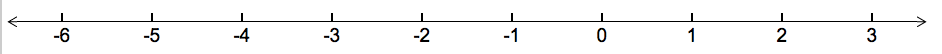The absolute value of a number is the number's distance from zero.

200

How can you rewrite 9in expanded form?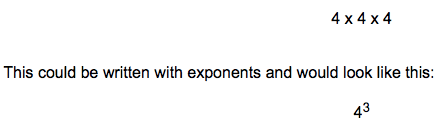200

Simplify the following expression: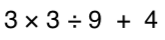200

14 + -12

https://www.khanacademy.org/math/arithmetic/arith-review-negative-numbers/arith-review-add-negatives-intro/v/adding-negative-numbers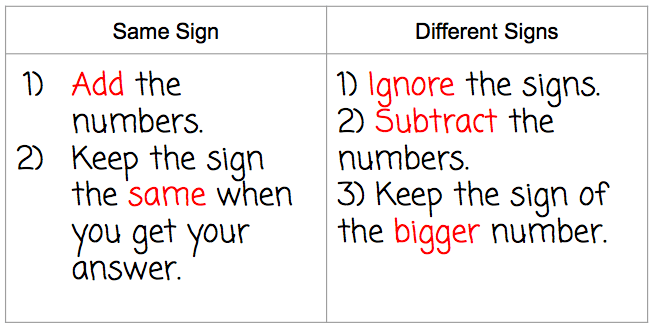200

5(x-3)

5x-15

200

What is 15% of 120

18

200

A train in Japan can travel 813.5 miles in 5 hrs.  Find the unit rate in miles per hour

167.7 mi / hr

300

Place the following in order from smallest number, to biggest number:

|-20|, 18, |12|, |-14|, 8

300

How can you rewrite the following in exponential form?

7 x 7 x 7 x 7 x 7300

3 + 42 * (3 + 2)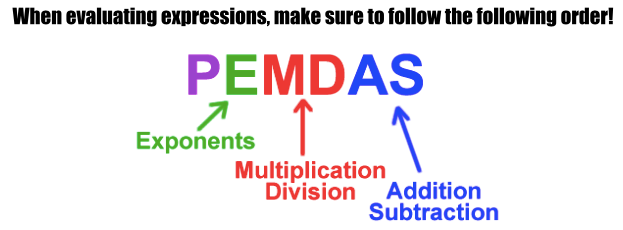300

-10 + 15300

7+9(2x-3)

18x-20

300

130% of 42

54.6

300

Arrange in fasted to slowest:

Abel reads 50 pages in 45 minutes; Brian reads 90 pages in 75 minutes; Charlie reads 175 pages in 2 hours

Charlie, Brian, Abel

400

What is:

|-7| + |-2|

The absolute value of a number is the number's distance from zero.

400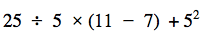400

-2 + -3 + 5

https://www.khanacademy.org/math/arithmetic/arith-review-negative-numbers/arith-review-add-negatives-intro/v/adding-negative-numbers400

-3(-x-10)

3x+30

400

8% of 540

43.2

400

An ice skater covered 1500 meters in 106 seconds.  What is her unit rate in meters per second

14.15 m per second

500

2 x |-5| + |4| - 14

Remember to use your order of operations.

500500

6 + (-2 + -5)

500

x + 10 -6(x-3x) +4

13x+14

500

70% of 478

334.6

500

Arrange from most expensive to least expensive if they buy lunch 5 days per week:

Alice spends \$3 per day; Betty spends \$25 every 2 weeks; Cindy spends \$75 per month (assume 4 weeks in a month)

Cindy, Alice, Betty

Click to zoom